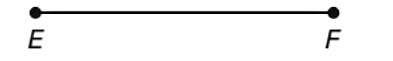Chapter P.3, Problem 46EElementary Geometry For College St...

7th Edition
Alexander + 2 others
ISBN: 9781337614085

Solutions

Chapter
SectionElementary Geometry For College St...

7th Edition
Alexander + 2 others
ISBN: 9781337614085
Textbook Problem

Use your compass and straightedge to bisect E F ¯ .To determine

To bisect:

EF¯ using the compass and straingtedge.

Explanation

Calculation:

Given,

Open the compass to a length greater than one-half of EF¯.

Using E as center of the arc, mark off an arc that extends both above and below the segment EF.

Now, with F as center and with the same length of radius mark off another arc, which also extends above and below EF¯ such that the points C and D are the intersection points of those two arches

Still sussing out bartleby?

Check out a sample textbook solution.

See a sample solution

The Solution to Your Study Problems

Bartleby provides explanations to thousands of textbook problems written by our experts, many with advanced degrees!

Get Started

Find more solutions based on key concepts